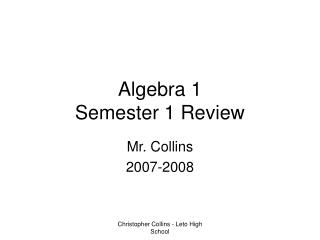# Algebra 1 Semester 1 Review - PowerPoint PPT PresentationDownload PresentationAlgebra 1 Semester 1 Review

Algebra 1 Semester 1 Review
Download Presentation## Algebra 1 Semester 1 Review

- - - - - - - - - - - - - - - - - - - - - - - - - - - E N D - - - - - - - - - - - - - - - - - - - - - - - - - - -
##### Presentation Transcript

1. Algebra 1Semester 1 Review Mr. Collins 2007-2008 Christopher Collins - Leto High School

2. 1. Evaluate and grid your answers. A. 12 Christopher Collins - Leto High School

3. 1. Evaluate and grid your answers. B. for x=6.25 1 Christopher Collins - Leto High School

4. 1. Evaluate and grid your answers. C. 1 5 / 4 Christopher Collins - Leto High School

5. 1. Evaluate and grid your answers. D. 7 / 2 Christopher Collins - Leto High School

6. 2. Give the slope of the line with equation Remember y = m x + b m = SLOPE The slope is 2! Christopher Collins - Leto High School

7. 3.Give the slope of the line with equation: slope is Christopher Collins - Leto High School

8. 4. Give the slope of the line with equation slope is Christopher Collins - Leto High School

9. -1/3 3 0 D. undefined E. 3/2 F. -2/3 5. Find the slope of each line. slope = m = Christopher Collins - Leto High School

10. 6. Find the y-intercept of the lines below. A. Remember that you can find the y-intercept by plugging in 0 for x and solving for y. Christopher Collins - Leto High School

11. 6. Find the y-intercept of the lines below. B. Remember that you can find the y-intercept by plugging in 0 for x and solving for y. Christopher Collins - Leto High School

12. 6. Find the y-intercept of the lines below. C. Remember that you can find the y-intercept by plugging in 0 for x and solving for y. Christopher Collins - Leto High School

13. 7. Find the x-intercept of the lines below. A. Remember that you can find the x-intercept by plugging in 0 for y and solving for x. Christopher Collins - Leto High School

14. 7. Find the x-intercept of the lines below. B. Remember that you can find the x-intercept by plugging in 0 for y and solving for x. Christopher Collins - Leto High School

15. 7. Find the x-intercept of the lines below. C. Remember that you can find the x-intercept by plugging in 0 for y and solving for x. Christopher Collins - Leto High School

16. A. Four more than twice a number. B. Four less than twice a number. C. Twice the sum of a number and four. D. Twice the difference of a number and four. E. The product of twice a number and four. F. Four less than twice a number. Choices: 1. 2. 3. 4. 5. 6. 7. 8. 8. Choose the algebraic expression for each verbal expression. (See the choices below.) MATCHING: Christopher Collins - Leto High School

17. 9. Use the distributive property to rewrite the expression: Answer choice C Christopher Collins - Leto High School

18. 10. Samuel and Joyce both bought CDs. Samuel bought 3x CDs and Joyce bought 2x-1 CDs. If each CD had a regular price of \$18 and a discount of \$2, then which expression would show the amount of discount for all of their CDs? The total number of cd’s The discount was \$2 per cd, Christopher Collins - Leto High School

19. What is the expression for the COST of their CDs, combined? (ignore tax) The total number of cd’s The price of cd’s were \$18-\$2 = \$16 apiece Christopher Collins - Leto High School

20. 11. Match the statement with the equation: (Choices are below) • Her salary is one dollar more than twice John’s salary. • B. John’s salary is twice hers plus \$1. • C. Twice John’s salary is the same as her salary plus one. • D. Twice her salary is the same as John’s salary plus \$1. Choices: 1. 2H=J+1 2. H=2J+1 3. 2J=H+1 4. J=2H+1 Christopher Collins - Leto High School

21. 12. Which expression represents the following sentence: Two pounds of nuts which cost x dollars a pound, and four pounds of chocolates which cost \$3 a pound costs \$20. • 2x + 3(20) = 4 • B. 2(x+4) = 20 • C. 2x+4(3)=20 • D. 2(x+12)= 20 Christopher Collins - Leto High School